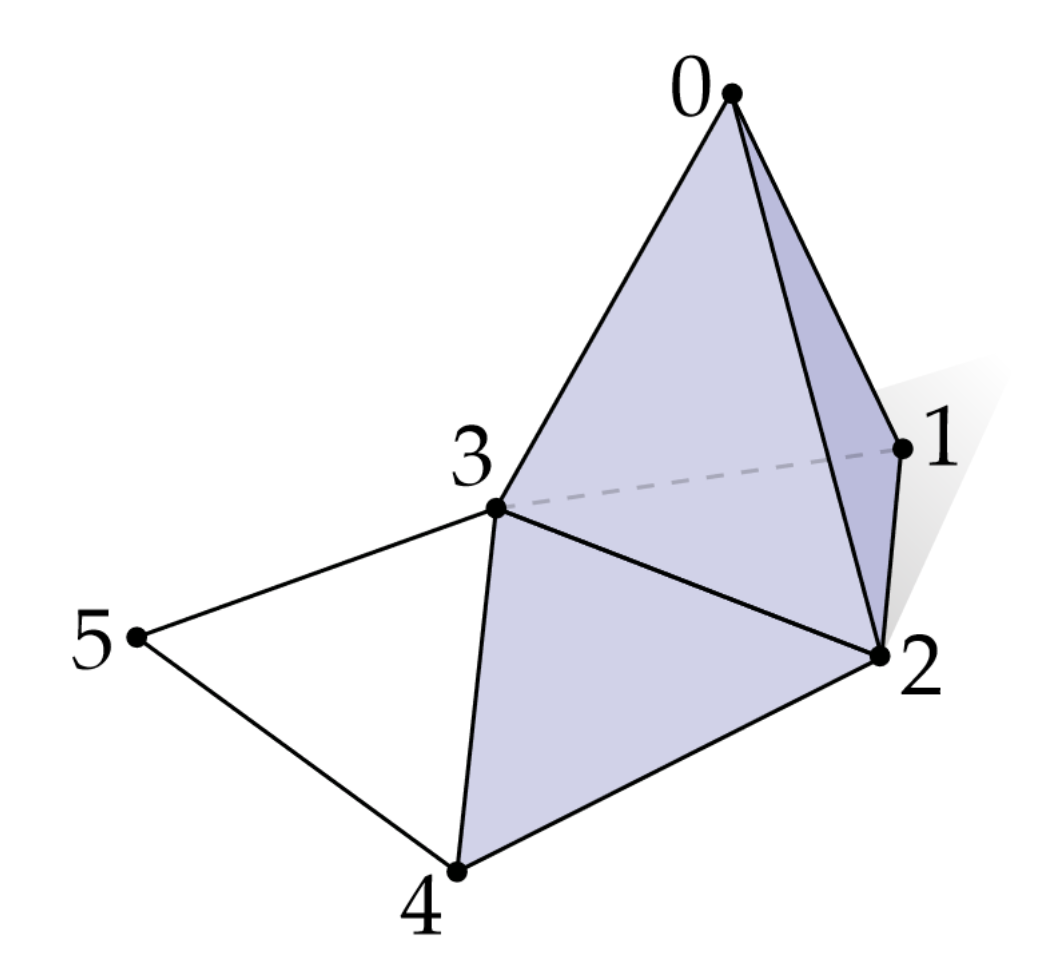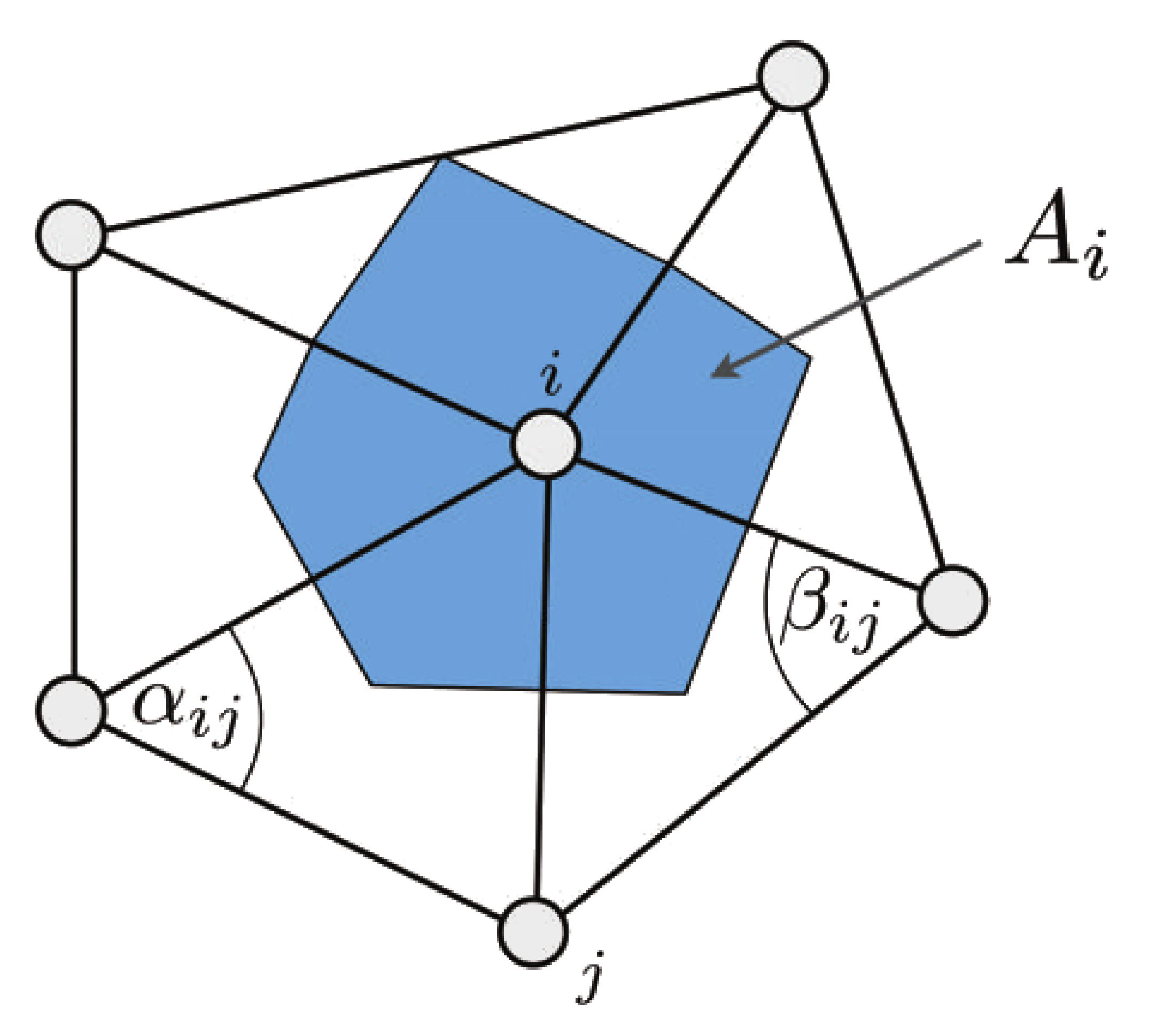# Definition: Laplacian Matrix for triangle meshesDefinition of the Laplacian matrix and Laplace operator on a triangular mesh. This is a cheat sheet / summary, I give an in depth explanation here.

## Laplacian matrix (triangle mesh)

Visually the matrix is symmetric and reflects the adjacency graph of our mesh, see a concrete illustration below and notice how vertex $$0$$ is connected to $$\{1, 2, 3\}$$; vertex $$1$$ to $$\{0, 2, 3\}$$ and so on:$$\newcommand{sumw}{ -\sum } \mathbf L_{ij} = \begin{bmatrix} \sumw{0} & w_{01} & w_{02} & w_{03} & 0 & 0 \\ w_{10} & \sumw{1} & w_{12} & w_{13} & 0 & 0 \\ w_{20} & w_{21} & \sumw{2} & w_{23} & w_{24} & 0 \\ w_{30} & w_{31} & w_{32} & \sumw{3} & w_{34} & w_{35} \\ 0 & 0 & w_{42} & w_{43} & \sumw{4} & w_{45} \\ 0 & 0 & 0 & w_{53} & w_{54} & \sumw{5} \end{bmatrix}$$

Formally, what is commonly called the Laplacian matrix $$\mathbf L$$ in the literature of geometry processing is:

$$\mathbf L_{ij} = \left \{ \begin{matrix} w_{ij} & = & \frac{1}{2} (\cot \alpha_{ij} + \cot \beta_{ij}) & \text{ if j adjacent to i} \\ & ~ & -\sum\limits_{{j \in \mathcal N(i)}} { w_{ij} } & \text{ when } j = i \\ & ~ & 0 &\text{ otherwise } \\ \end{matrix} \right .$$

• $$\mathbf L \in \mathbb R^{n \times n}$$ with $$n$$ the number of vertices of the mesh
• $$\mathbf L_{ij}$$ is a single element of the matrix
• The row $$i$$ and column $$j$$ of the matrix represent vertex indices as well
• $${j \in \mathcal N(i)}$$ is the list of vertices directly adjacent to the vertex $$i$$
• Each row of $$\mathbf L$$ contains the list of vertices weights adjacent to $$i$$
• $$\mathbf L$$ is symmetric positive semi-definite
• $$\cot \alpha_{ij}$$ and $$\cot \beta_{ij}$$ is the evaluation of the cotangent function at $$x= \alpha_{ij}$$ and $$y = \beta_{ij}$$ (c.f. figure below)$$\cot( \theta ) = \frac{\cos \theta}{\sin \theta} =\frac{\vec u . \vec v}{ \|\vec u \times \vec v\|} = \frac{\|\vec u\|.\|\vec v\| cos(\theta) }{\|\vec u\|.\|\vec v\| sin(\theta)}$$

Remark: the general Laplacian matrix as defined in graph theory (as opposed to geometry processing like in here) is usually defined as $$-\mathbf L$$

## Discrete Laplace operator

On the other hand the Laplacian *operator* is defined as $$\mathbf {\Delta f} = {\mathbf M}^{-1} \mathbf L$$ with the Mass matrix $$\mathbf M$$, a diagonal matrix that stores the cell area (blue area on the figure) of each vertex:

$$\mathbf M^{-1} = \begin{bmatrix} \frac{1}{A_0} & 0 & 0 \\ 0 & \ddots & 0 \\ 0 & 0 & \frac{1}{A_n}\\ \end{bmatrix}$$

$$A_i = \frac{1}{3} \sum\limits_{{T_j \in \mathcal N(i)}} {area(T_j)}$$

• $$T_j \in \mathcal N(i)$$ list of triangles adjacent to $$i$$
• $$A_i$$ can also be computed with mixed voronoi area.

## Code

See the [C++ code ] to build the laplacian matrix with cotan weights (get_laplacian() procedure)

If your mesh is represented with an half-edge data structure (each vertex knows its direct neighbours) the pseudo code to compute $$\mathbf L$$ is:


// angle(i,j,t) -> angle at the vertex opposite to the edge (i,j)
for(int i : vertices) {
for(int j : one_ring(i)) {
sum = 0;
for(int t : triangle_on_edge(i,j))
{
w = cot(angle(i,j,t));
L(i,j) = w;
sum += w;
}
L(i,i) = -sum;
}
}


On the other hand the Laplacian $$\mathbf L$$ may be built by summing together contributions for each triangle, this way only the list of triangles is needed:

for(triangle t : triangles)
{
for(edge i,j : t)
{
w = cot(angle(i,j,t));
L(i,j) += w;
L(j,i) += w;
L(i,i) -= w;
L(j,j) -= w;
}
}